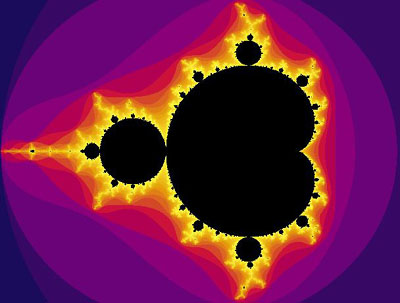A# Mandelbrot setThe Mandelbrot set is the best known fractal and one of the most complex and beautiful mathematical objects known. It was discovered by Benoît Mandelbrot in 1980 and named after him by Adrien Douady and J. Hubbard in 1982.

The Mandelbrot set is produced by the incredibly simple iteration formula:

zn+1 = zn2 + c,

where z and c are complex numbers and z0 = 0. This can be written without complex numbers as

xn+1 = xn2 - yn2 + a, and
yn+1 = 2xnyn + b

where z = (x, y) and c = (a, b). The Mandelbrot set consists of all the points on the complex plane (see Argand diagram) for which the function z2 + c doesn't diverge under iteration.

A computer is essential for carrying out the necessary calculations and for producing pictures of this remarkable structure. For the purposes of computation, the complex plane is broken down into pixels (picture elements), the coordinates of each of which supply the constant c in z2 + c. For each pixel (value of c) the function is iterated. If the function either rapidly diverges (blows up) or rapidly converges (collapses), the pixel is left black. If the function is more indecisive about which way it is heading, it is allowed to iterate longer. In some cases the iterations could go on for a very long time before it became clear that the function would ultimately diverge, so a limit is established, known as the depth, beyond which iterations are stopped. If the depth is reached without divergence, the corresponding pixel is left black as though it were in the set. At locations where divergence is indicated prior to hitting the limit, the pixel is displayed according to a scale that represents how many iterations are needed to show divergence.

The whole Mandelbrot set lies within a circle of radius 2.5 centered at the origin of the complex plane. Although finite in area, the Set has a boundary that is infinitely long and has a Hausdorff dimension of 2. The overall appearance of the Mandelbrot set is that of a series of disks. These disks have irregular borders and decrease in size heading out along the negative real axis; moreover, the ratio of the diameter of one disk to the next approaches a constant. More complex shapes branch out from the disks. One region of the Mandelbrot set containing spiral shapes is known as Seahorse Valley because it resembles a seahorse's tail. A computer can be used, like a microscope, to zoom in on different parts of the set. This reveals that, although the shape is infinitely complex, it also displays self-similarity with regions that look like the outline of the entire set.

The Mandelbrot set also exhibits symmetry on different levels. It is identically symmetrical about the real axis, and almost symmetrical at smaller scales. This kind of "near-but-not-quite" symmetry is one of the most unexpected properties to find in an object generated from such a simple formula and process. The Mandelbrot set was created by Mandelbrot as an index to the Julia sets. Each point in the complex plane corresponds to a different Julia set, and those points within the Mandelbrot set correspond precisely to the connected Julia sets.